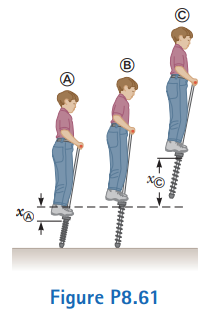# Problem: A child’s pogo stick (Fig. P8.61) stores energy in a spring with a force constant of 2.50 x  104 N/m. At position A (xA = - 0.100  m), the spring compression is a maximum and the child is momentarily at rest. At position B (xB = 0), the spring is relaxed and the child is moving upward. At position C, the child is again momentarily at rest at the top of the jump. The combined mass of child and pogo stick is 25.0 kg. Although the boy must lean forward to remain balanced, the angle is small, so let’s assume the pogo stick is vertical. Also assume the boy does not bend his legs during the motion.(a) Calculate the total energy of the child–stick–Earth system, taking both gravitational and elastic potential energies as zero for x = 0.(b) Determine xC.(c) Calculate the speed of the child at x = 0.(d) Determine the value of x for which the kinetic energy of the system is a maximum.(e) Calculate the child’s maximum upward speed.

⚠️Our tutors found the solution shown to be helpful for the problem you're searching for. We don't have the exact solution yet.

###### Problem Details

A child’s pogo stick (Fig. P8.61) stores energy in a spring with a force constant of 2.50 x  104 N/m. At position A (xA = - 0.100  m), the spring compression is a maximum and the child is momentarily at rest. At position B (xB = 0), the spring is relaxed and the child is moving upward. At position C, the child is again momentarily at rest at the top of the jump. The combined mass of child and pogo stick is 25.0 kg. Although the boy must lean forward to remain balanced, the angle is small, so let’s assume the pogo stick is vertical. Also assume the boy does not bend his legs during the motion.

(a) Calculate the total energy of the child–stick–Earth system, taking both gravitational and elastic potential energies as zero for x = 0.
(b) Determine xC.
(c) Calculate the speed of the child at x = 0.
(d) Determine the value of x for which the kinetic energy of the system is a maximum.
(e) Calculate the child’s maximum upward speed.